# Easiest colleges to get into in Iowa

Top 10 colleges in Iowa with the highest acceptance rates
What are the easiest colleges to get into in Iowa? We've got you covered. We've compiled a national college database and have created a list of the easiest colleges to get into in Iowa below. These are the easiest 4 year colleges to get into in Iowa that have the highest acceptance rates, and are more likely to accept low GPAs, GEDs, and everything in between. There are tons of good colleges below that accept more applicants, and we also share their average SAT and ACT scores so that you can see where you're most competitive. Read on to find out more.

## Loras College acceptance rate

Loras College acceptance rate is 92.7%.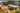The average SAT score for Loras College is 1099.

• SAT composite: 1099
• SAT math: 560

The average ACT score for Loras College is 23.

## Iowa State University acceptance rate

Iowa State University acceptance rate is 92.1%.The average SAT score for Iowa State University is 1220.

• SAT composite: 1220
• SAT math: 625

The average ACT score for Iowa State University is 25.

## Univeristy of Iowa acceptance rate

Univeristy of Iowa acceptance rate is 82.6%.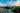The average SAT score for Univeristy of Iowa is 1235.

• SAT composite: 1235
• SAT math: 625

The average ACT score for Univeristy of Iowa is 26.

## Simpson College acceptance rate

Simpson College acceptance rate is 82.5%.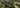The average SAT score for Simpson College is 1180.

• SAT composite: 1180
• SAT math: 590

The average ACT score for Simpson College is 24.

## University of Northern Iowa acceptance rate

University of Northern Iowa acceptance rate is 80.7%.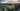The average SAT score for University of Northern Iowa is 1140.

• SAT composite: 1140
• SAT math: 570

The average ACT score for University of Northern Iowa is 23.

## Wartburg College acceptance rate

Wartburg College acceptance rate is 75.7%.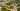The average SAT score for Wartburg College is 1090.

• SAT composite: 1090
• SAT math: 550

The average ACT score for Wartburg College is 23.

## Dordt College acceptance rate

Dordt College acceptance rate is 75.4%.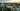The average SAT score for Dordt College is 1230.

• SAT composite: 1230
• SAT math: 630

The average ACT score for Dordt College is 25.

## Waldorf College acceptance rate

Waldorf College acceptance rate is 71.9%.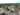The average SAT score for Waldorf College is 1030.

• SAT composite: 1030
• SAT math: 510

The average ACT score for Waldorf College is 21.

## Drake University acceptance rate

Drake University acceptance rate is 67.7%.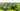The average SAT score for Drake University is 1248.

• SAT composite: 1248
• SAT math: 620

The average ACT score for Drake University is 27.

## Luther College acceptance rate

Luther College acceptance rate is 65.1%.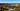The average SAT score for Luther College is 1165.

• SAT composite: 1165
• SAT math: 590